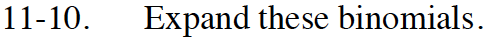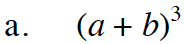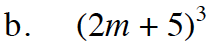### Home > INT3 > Chapter 11 > Lesson 11.1.1 > Problem11-10

11-10.
1. Expand these binomials. Homework Help ✎

1. (a + b)3

2. (2m + 5)3Review the Math Notes on the Binomial Theorem in lesson 10.3.3. Remember, you can also use Pascal's Triangle to help you here.

(a + b)3 = 3C3a3b0 + 3C2a2b1 + 3C1a1b2 + 3C0a0b3Use the result from part (a).
a = 2m; b = 5. Simplify.